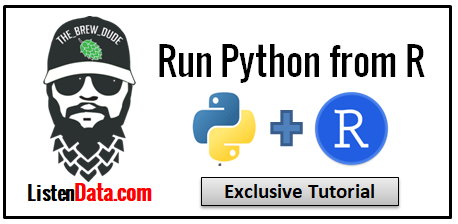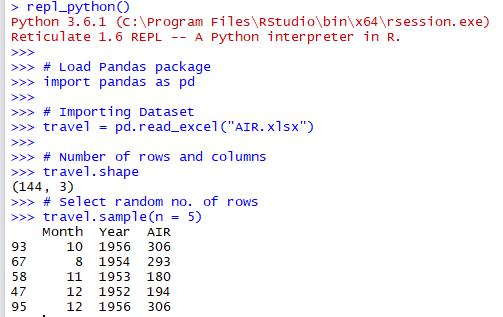### Run Python from R

This article explains how to call or run python from R. Both the tools have its own advantages and disadvantages. It's always a good idea to use the best packages and functions from both the tools and combine it. In data science world, these tools have a good market share in terms of usage. R is mainly known for data analysis, statistical modeling and visualization. While python is popular for deep learning and natural language processing.

In recent KDnuggets Analytics software survey poll, Python and R were ranked top 2 tools for data science and machine learning. If you really want to boost your career in data science world, these are the languages you need to focus on.Combine Python and R

RStudio developed a package called reticulate which provides a medium to run Python packages and functions from R.

Run the command below to get this package installed and imported to your system.
# Install reticulate package
install.packages("reticulate")

library(reticulate)

Check whether Python is available on your system
py_available()
It returns TRUE/FALSE. If it is TRUE, it means python is installed on your system.

Import a python module within R

You can use the function import( ) to import a particular package or module.
os <- import("os")
os\$getcwd()
The above program returns working directory.
``` "C:\\Users\\DELL\\Documents"
```

You can use listdir( ) function from os package to see all the files in working directory
os\$listdir()
```  ".conda"                       ".gitignore"                   ".httr-oauth"
 ".matplotlib"                  ".RData"                       ".RDataTmp"
 ".Rhistory"                    "1.pdf"                        "12.pdf"
 "122.pdf"                      "124.pdf"                      "13.pdf"
 "1403.2805.pdf"                "2.pdf"                        "3.pdf"
 "AIR.xlsx"                     "app.r"                        "Apps"
 "articles.csv"                 "Attrition_Telecom.xlsx"       "AUC.R"
```

Install Python Package

Step 1 : Create a new environment
conda_create("r-reticulate")
Step 2 : Install a package within a conda environment
conda_install("r-reticulate", "numpy")
Since numpy is already installed, you don't need to install it again. The above example is just for demonstration.

Step 3 : Load the package
numpy <- import("numpy")

Working with numpy array

Let's create a sample numpy array
y <- array(1:4, c(2, 2))
x <- numpy\$array(y)
```     [,1] [,2]
[1,]    1    3
[2,]    2    4
```

Transpose the above array
numpy\$transpose(x)
```    [,1] [,2]
[1,]    1    2
[2,]    3    4
```

Eigenvalues and eigen vectors
numpy\$linalg\$eig(x)
```[]
 -0.3722813  5.3722813

[]
[,1]       [,2]
[1,] -0.9093767 -0.5657675
[2,]  0.4159736 -0.8245648
```

Mathematical Functions
numpy\$sqrt(x)
numpy\$exp(x)

Working with Python interactively

You can create an interactive Python console within R session. Objects you create within Python are available to your R session (and vice-versa).

By using `repl_python()` function, you can make it interactive. Download the dataset used in the program below.
repl_python()

import pandas as pd

# Importing Dataset

# Number of rows and columns
travel.shape

# Select random no. of rows
travel.sample(n = 10)

# Group By
travel.groupby("Year").AIR.mean()

# Filter
t = travel.loc[(travel.Month >= 6) & (travel.Year >= 1955),:]

exit
Note : You need to enter exit to return to the R environment.Run Python from R

How to access objects created in python from R

You can use the py object to access objects created within python.
summary(py\$t)
In this case, I am using R's summary( ) function and accessing dataframe t which was created in python. Similarly, you can create line plot using ggplot2 package.
# Line chart using ggplot2
library(ggplot2)
ggplot(py\$t, aes(AIR, Year)) + geom_line()

How to access objects created in R from Python

You can use the r object to accomplish this task.

1. Let's create a object in R
2. Use the R created object within Python REPL
repl_python()
import pandas as pd
r.mydata.describe()
pd.isnull(r.mydata.speed)
exit

Building Logistic Regression Model using sklearn package

The sklearn package is one of the most popular package for machine learning in python. It supports various statistical and machine learning algorithms.
repl_python()

from sklearn import datasets
from sklearn.linear_model import LogisticRegression

# Developing logit model
model = LogisticRegression()
model.fit(iris.data, iris.target)

# Scoring
actual = iris.target
predicted = model.predict(iris.data)

# Performance Metrics
print(metrics.classification_report(actual, predicted))
print(metrics.confusion_matrix(actual, predicted))

Other Useful Functions

To see configuration of python

Run the py_config( ) command to find the version of python installed on your system.It also shows details about anaconda and numpy.
py_config()
```python:         C:\Users\DELL\ANACON~1\python.exe
libpython:      C:/Users/DELL/ANACON~1/python36.dll
pythonhome:     C:\Users\DELL\ANACON~1
version:        3.6.1 |Anaconda 4.4.0 (64-bit)| (default, May 11 2017, 13:25:24) [MSC v.1900 64 bit (AMD64)]
Architecture:   64bit
numpy:          C:\Users\DELL\ANACON~1\lib\site-packages\numpy
numpy_version:  1.14.2```

To check whether a particular package is installed

In the following program, we are checking whether pandas package is installed or not.
py_module_available("pandas")Related Posts
8 Responses to "Run Python from R"
1.The py_config() gives the version of Anakonda, I think you mentioned that it is giving R version installed on system..

2.Great package and great tutorial. This will be particularly helpful for the R enthusiasts looking into deep learning I would imagine

3.This comment has been removed by the author.

4.5.This comment has been removed by the author.

6.This comment has been removed by a blog administrator.

7.Thanks for sharing this post. Your post is really very helpful its students. python Online course

8.Good Post! you have furnished the right information that will be useful to anyone at all time. Thanks for sharing your Ideas.

Python Training
Microstrategy Training